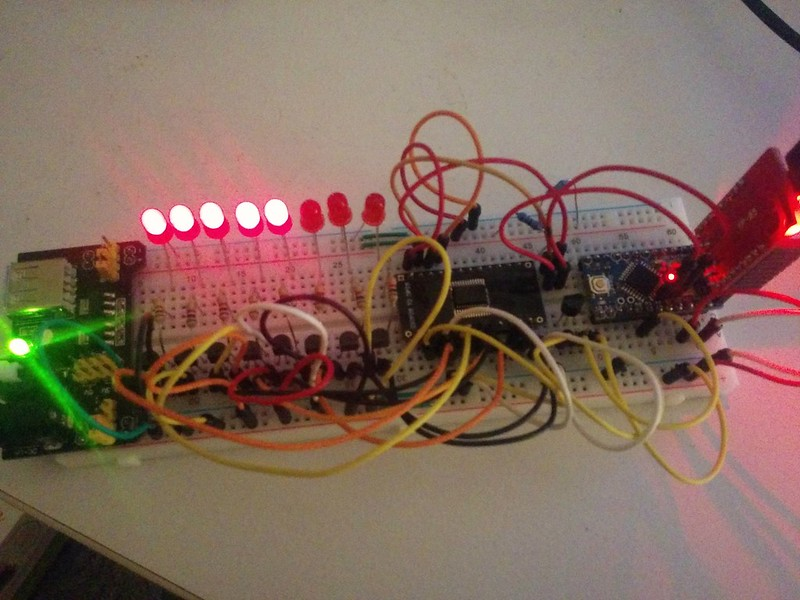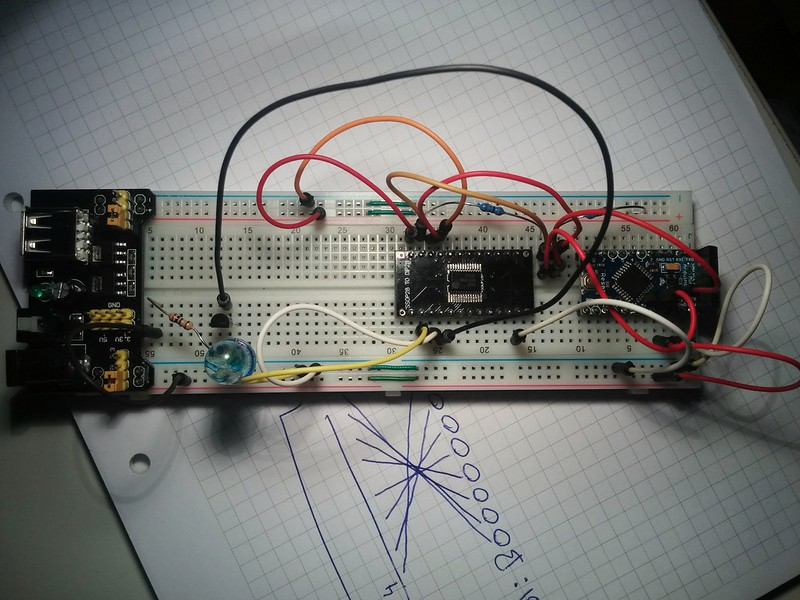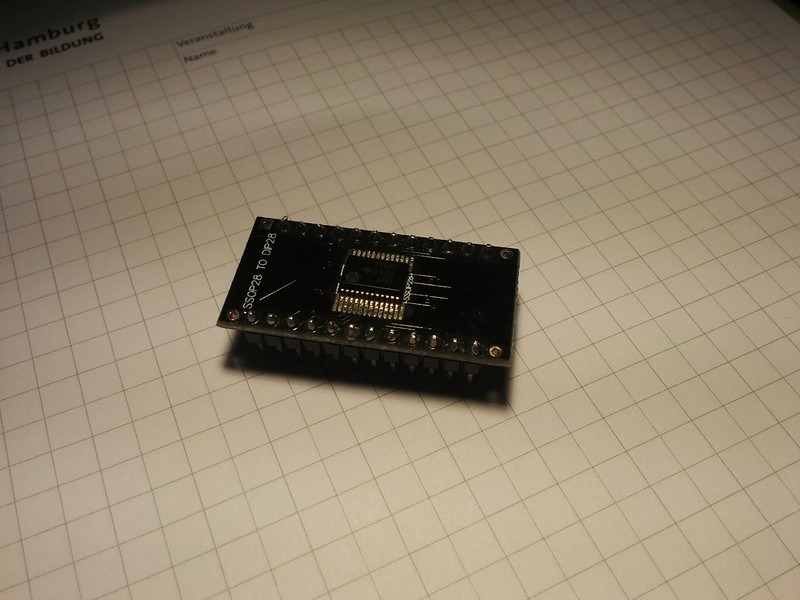## Arduino PCF8575 8xLED LM35 ThermometerMy code:

````#include <Wire.h>`

`// Set I2C address`
`int address = 0x20;`

`int samples = 2;`
`int collectDelay = 1000;`
`int ledPin = 13;`
`int tempPin = 0;`

`float tempC = 0;`

`void setup() {`
`  pinMode(ledPin, OUTPUT);`
`  Wire.begin();`
`  pf575_write(word(B11111111,B11111111));`
`  delay(200);`
`  pf575_write(word(B00000000,B11111111));`
`  delay(200);`
`  pf575_write(word(B11111111,B11111111));`
`  delay(200);`
`  pf575_write(word(B00000000,B11111111));`
`  delay(200);`
`  pf575_write(word(B11111111,B11111111));`
`  delay(1000);`
`}`

`void loop() {`
`  tempC = 0;`
`  for(int i = 0; i <= (samples - 1); i++) {`
`    tempC = tempC + ((5.0 * analogRead(tempPin) * 100.0) / 1024.0);`
`    digitalWrite(ledPin, HIGH);`
`    delay((collectDelay / 2));`
`    digitalWrite(ledPin, LOW);`
`    delay((collectDelay / 2));`
`  }`
`  tempC = tempC / (float)samples;`
`  if(tempC > 21) {`
`    pf575_write(word(B11111110,B11111111));`
`  }`
`  if(tempC > 23) {`
`    pf575_write(word(B11111100,B11111111));`
`  }`
`  if(tempC > 25) {`
`    pf575_write(word(B11111000,B11111111));`
`  }`
`  if(tempC > 27) {`
`    pf575_write(word(B11110000,B11111111));`
`  }`
`  if(tempC > 29) {`
`    pf575_write(word(B11100000,B11111111));`
`  }`
`  if(tempC > 31) {`
`    pf575_write(word(B11000000,B11111111));`
`  }`
`  if(tempC > 33) {`
`    pf575_write(word(B10000000,B11111111));`
`  }`
`  if(tempC > 35) {`
`    pf575_write(word(B00000000,B11111111));`
`  }`
`}`

`void pf575_write(uint16_t data) {`
`  Wire.beginTransmission(address);`
`  Wire.write(highByte(data));`
`  Wire.write(lowByte(data));`
`  Wire.endTransmission();`
`}````

## Arduino PF575 I²C I/O port expander blink example

I got some TI PCF8575 I²C I/O expander devices some years ago and never gave them a try. Yesterday I did... In the title they are named PF575, this is because this is what is printed on the device.

I wrote code for making a LED blink using the I/O expander as simple as possible.

Here you see my setup on a breadboard. On the left is the LED connected via a PNP transistor for voltage control.I only had 24-SSOP packages so I needed to solder the PCF8575 to a DIP adapter for making it breadboard friendly.My code:

````#include <Wire.h>`

`/**`
` *  Arduino PF575/PCF8575 I2C I/O port exapander LED blink example`
` *`
` *  Setup:`
` *`
` *  1. Connect A0, A1 and A2 to GND to set the address to 0x20.`
` *  2. Connect SDL and SCL to the Arduino's I2C bus.`
` *  3. Connect a LED to the P0 port of the I2C exapander.`
` *     In my setup I am using a PNP Transistor connected to 5V unsing`
` *     an resistor, the LED and the Arduino to make sure the LED gets`
` *     a current from Vcc and not from the I2C exapander port.`
` *`
` *  This code is trying to explain how it works as simple as possible.`
` *  More detailed examples are found on the web. Search for pcf8575 and`
` *  you will find what you want.`
`*/`

`// Set I2C address`
`int address = 0x20;`

`void setup(){`
`  Wire.begin();`
`  // Set all ports as output`
`  pf575_write(word(B11111111,B11111111));`
`}`

`void loop(){`
`  // Set port P0 on`
`  pf575_write(word(B00000000,B00000001));`
`  delay(1000);`
`  // Set port P0 off`
`  pf575_write(word(B00000000,B00000000));`
`  delay(1000);`
`}`

`// Function for writing two Bytes to the I2C expander device`
`void pf575_write(uint16_t data) {`
`  Wire.beginTransmission(address);`
`  Wire.write(lowByte(data));`
`  Wire.write(highByte(data));`
`  Wire.endTransmission();`
`}````